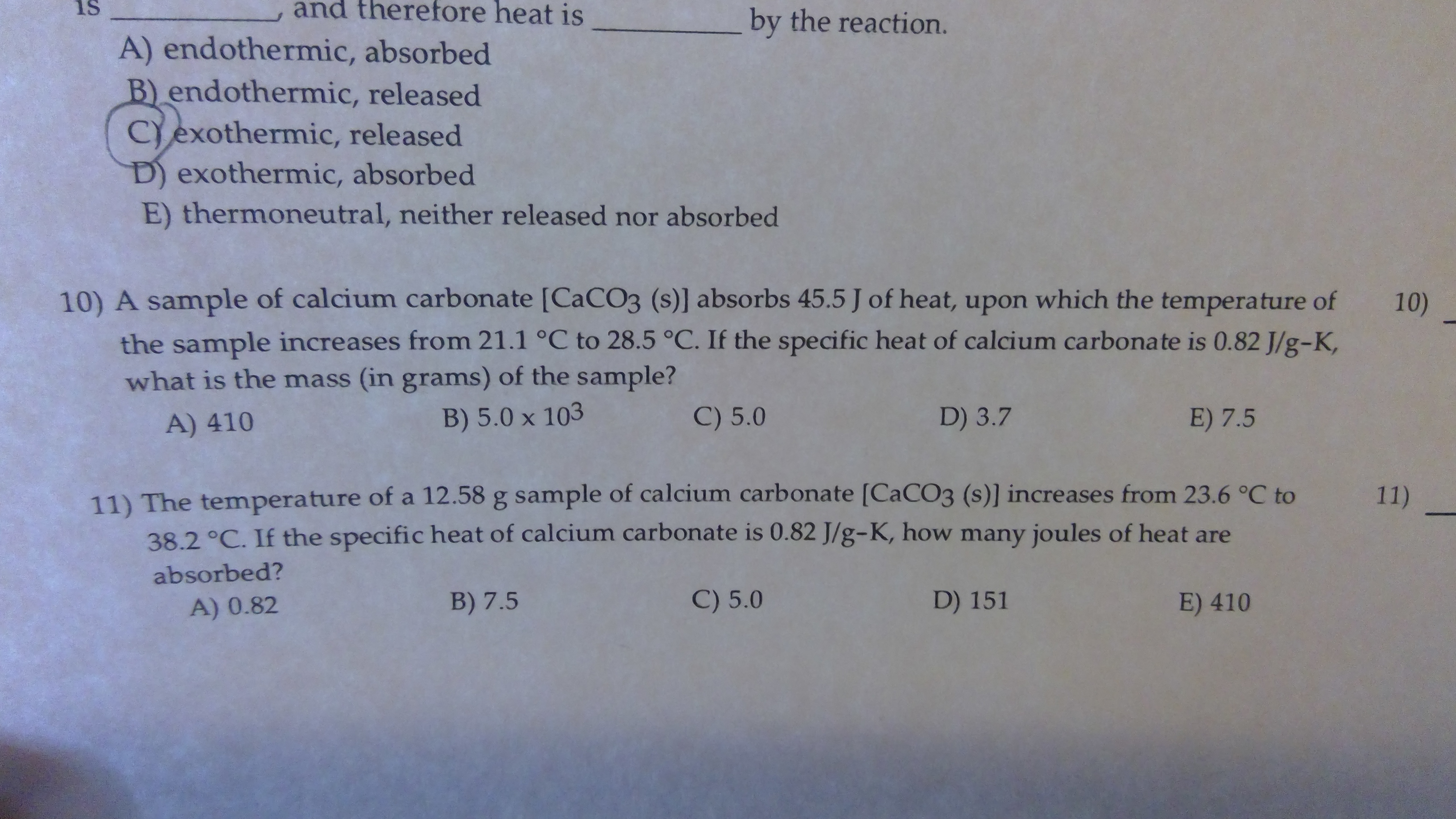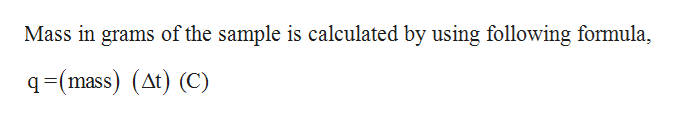# and therefore heat isisby the reaction.A) endothermic, absorbedB) endothermic, releasedCexothermic, releasedD) exothermic, absorbedE) thermoneutral, neither released nor absorbed10) A sample of calcium carbonate [CaCO3 (s)] absorbs 45.5 J of heat, upon which the temperature of10)the sample increases from 21.1 °C to 28.5 °C. If the specific heat of calcium carbonate is 0.82 J/g-K,what is the mass (in grams) of the sample?C) 5.0D) 3.7E) 7.5B) 5.0 x 103A) 41011) The temperatu re of a 12.58 g sample of calcium carbonate [CaCO3 (s)] incre ases from 23.6 °C to38.2 °C. If the specific heat of calcium carbonate is 0.82 J/g-K, how many joules of heat are11)absorbed?C) 5.0D) 151B) 7.5E) 410A) 0.82

Question

How would I solve for the mass ( in grams )help_outlineImage Transcriptioncloseand therefore heat is is by the reaction. A) endothermic, absorbed B) endothermic, released Cexothermic, released D) exothermic, absorbed E) thermoneutral, neither released nor absorbed 10) A sample of calcium carbonate [CaCO3 (s)] absorbs 45.5 J of heat, upon which the temperature of 10) the sample increases from 21.1 °C to 28.5 °C. If the specific heat of calcium carbonate is 0.82 J/g-K, what is the mass (in grams) of the sample? C) 5.0 D) 3.7 E) 7.5 B) 5.0 x 103 A) 410 11) The temperatu re of a 12.58 g sample of calcium carbonate [CaCO3 (s)] incre ases from 23.6 °C to 38.2 °C. If the specific heat of calcium carbonate is 0.82 J/g-K, how many joules of heat are 11) absorbed? C) 5.0 D) 151 B) 7.5 E) 410 A) 0.82 fullscreen
check_circleExpert Solution
Step 1

Given,

Heat absorbed = 45.5 J

Mass of sample = ?

Specific heat of sample = 0.82 J/g K

Change in ...help_outlineImage TranscriptioncloseMass in grams of the sample is calculated by using following formula =(mass) (At) (C) fullscreen

### Want to see the full answer?

See Solution

#### Want to see this answer and more?

Solutions are written by subject experts who are available 24/7. Questions are typically answered within 1 hour*

See Solution
*Response times may vary by subject and question
Tagged in

### General Chemistry